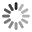The best jokes and joke writers!## Girls Are Evil

Girls = time * money

time = money, therefore: Girls = money * money (*)

But we know that money is a root of all evil, thus: m>

Taking into account (*), we have: Girls = sqrt(evil)*sqrt(evil)

And finally: Girls = |evil| Thus, Girls are the absolute evil!

## Numbers Equal Zero

Theorem : All numbers are equal to zero.

Proof: Suppose that a=b. Then a = b a^2 = ab a^2 - b^2 = ab - b^2 (a + b)(a - b) = b(a - b) a + b = b a = 0 Furthermore if a + b = b, and a = b, then b + b = b, and 2b = b, which mean that 2 = 1.

## Bird Math

Q: What kind of math do birds like?

A: Owlgebra.

## Three is Equal to Four

Theorem: 3=4

Proof: Suppose: a + b = c This can also be written as: 4a - 3a + 4b - 3b = 4c - 3c After reorganizing: 4a + 4b - 4c = 3a + 3b - 3c Take the constants out of the brackets: 4 * (a+b-c) = 3 * (a+b-c) Remove the same term left and right: 4 = 3

## Numbers Class

Q: 1,2,3,4 & 5 are sitting in class, why did 3 get kicked out?

A: He was being mean.